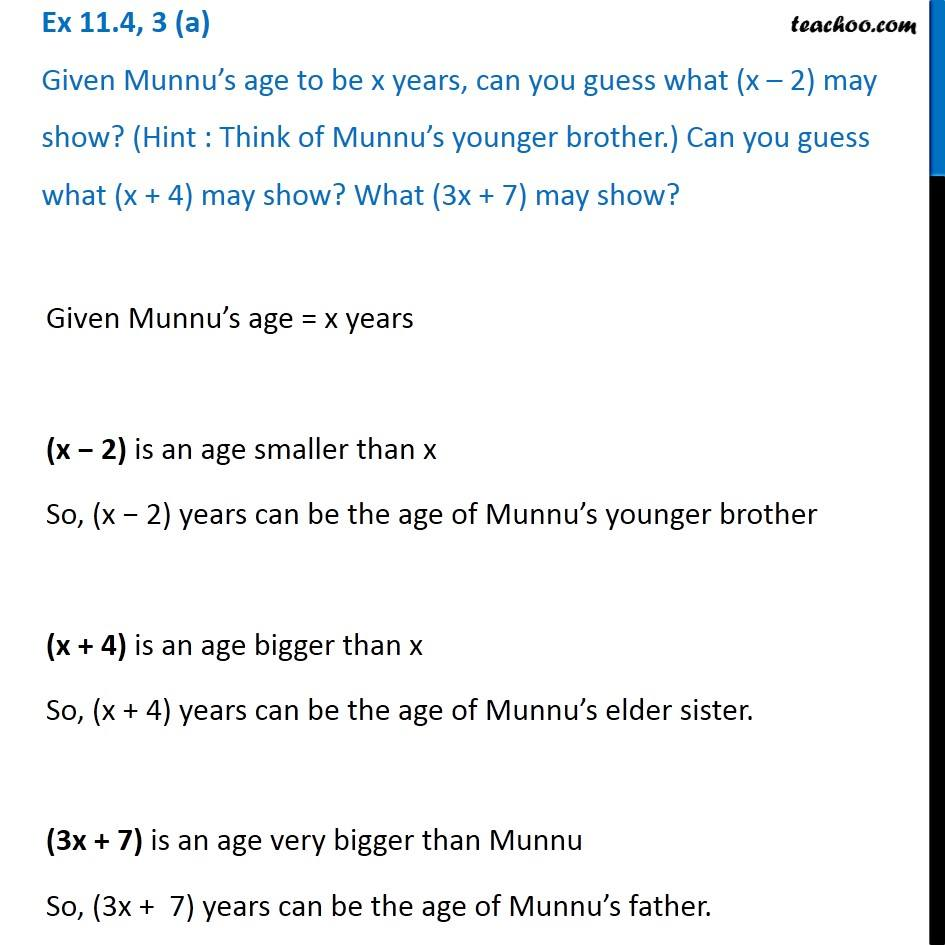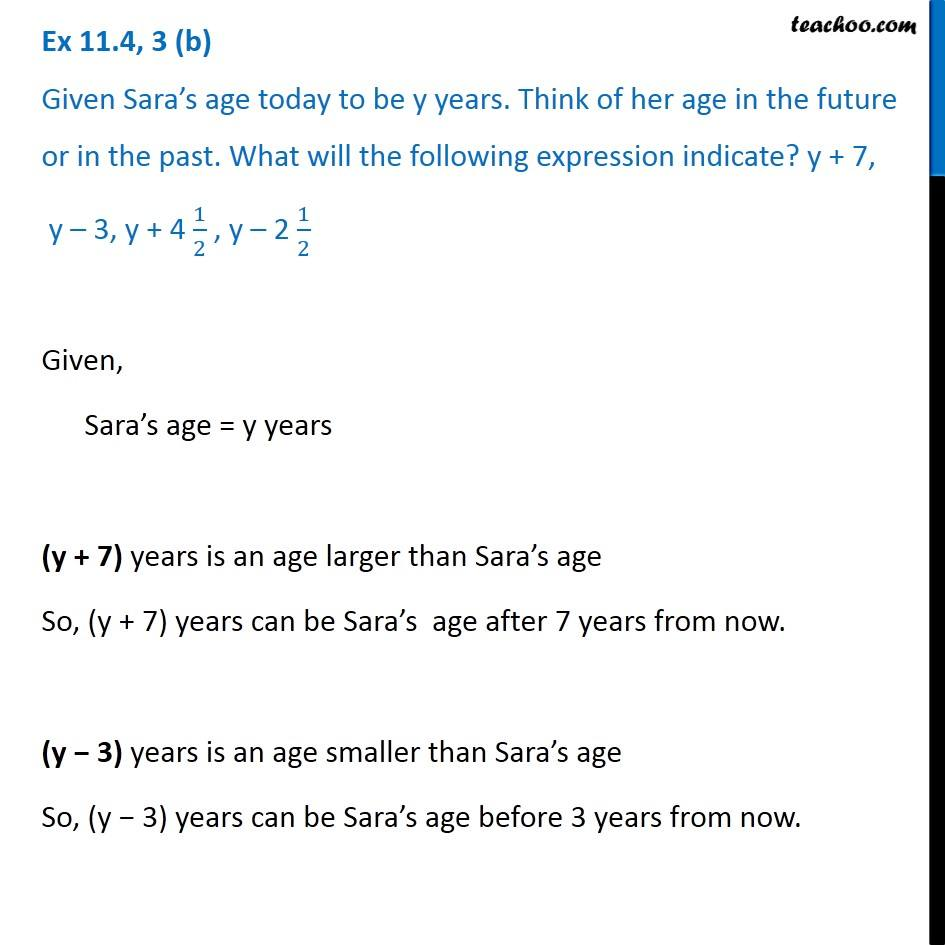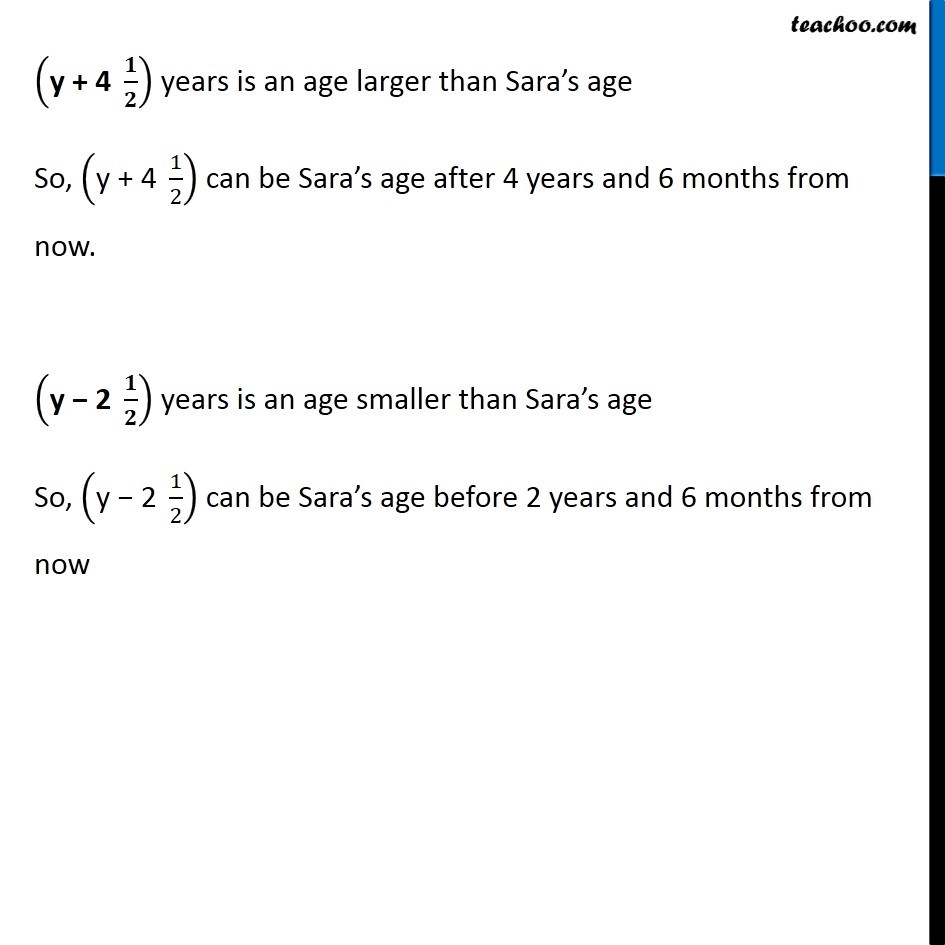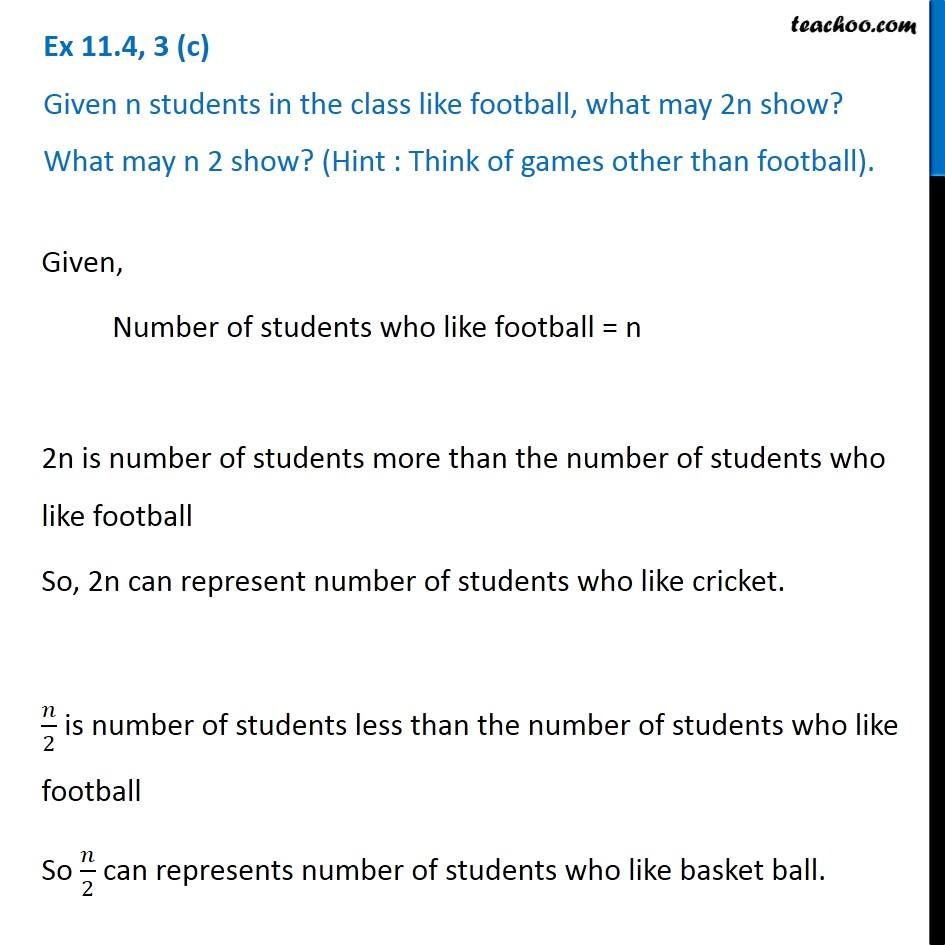Subscribe to our Youtube Channel - https://you.tube/teachoo

1. Chapter 11 Class 6 Algebra
2. Serial order wise
3. Ex 11.4

Transcript

Ex 11.4, 3 (a) Given Munnu’s age to be x years, can you guess what (x – 2) may show? (Hint : Think of Munnu’s younger brother.) Can you guess what (x + 4) may show? What (3x + 7) may show? Given Munnu’s age = x years (x − 2) is an age smaller than x So, (x − 2) years can be the age of Munnu’s younger brother (x + 4) is an age bigger than x So, (x + 4) years can be the age of Munnu’s elder sister. (3x + 7) is an age very bigger than Munnu So, (3x + 7) years can be the age of Munnu’s father. Ex 11.4, 3 (b) Given Sara’s age today to be y years. Think of her age in the future or in the past. What will the following expression indicate? y + 7, y – 3, y + 4 1/2 , y – 2 1/2Given, Sara’s age = y years (y + 7) years is an age larger than Sara’s age So, (y + 7) years can be Sara’s age after 7 years from now. (y − 3) years is an age smaller than Sara’s age So, (y − 3) years can be Sara’s age before 3 years from now. ("y + 4 " 𝟏/𝟐) years is an age larger than Sara’s age So, ("y + 4 " 1/2) can be Sara’s age after 4 years and 6 months from now. ("y − 2 " 𝟏/𝟐) years is an age smaller than Sara’s age So, ("y − 2 " 1/2) can be Sara’s age before 2 years and 6 months from now Ex 11.4, 3 (c) Given n students in the class like football, what may 2n show? What may n 2 show? (Hint : Think of games other than football). Given, Number of students who like football = n 2n is number of students more than the number of students who like football So, 2n can represent number of students who like cricket. 𝑛/2 is number of students less than the number of students who like football So 𝑛/2 can represents number of students who like basket ball.

Ex 11.4

Chapter 11 Class 6 Algebra
Serial order wise